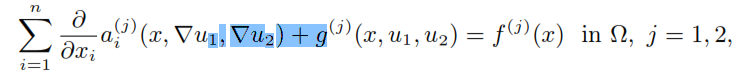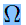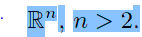##### Effects of Hall Current and Rotation on Unsteady MHD Natural Convection Flow Past a Vertical Flat Plate with Ramped Wall Temperature and Heat Absorption

K. K. Pandit, D. Sarma, A. K. Deka

Journal of Advances in Mathematics and Computer Science, Page 1-26
DOI: 10.9734/BJMCS/2016/27221

In the present study, the effects of Hall current and rotation on an unsteady Magneto-Hydrodynamic (MHD) free convection heat and mass transfer of an electrically conducting, viscous, incompressible and heat absorbing fluid flow past a vertical infinite flat plate embedded in non-Darcy porous medium is investigated. The flow is induced by a general time-dependent movement of the vertical plate, and the cases of ramped temperature and isothermal plates are studied. Exact solution of the governing time-dependent boundary layer equations for the momentum, energy and concentration were obtained in closed form by using Laplace Transform technique. Expressions for skin friction due to primary and secondary flows and Nusselt number are derived for both ramped temperature and isothermal plates. Expression for Sherwood number is also derived. Some applications of practical interest for different types of plate motions viz. plate moving with uniform velocity, plate moving with uniform acceleration and plate moving with periodic acceleration are discussed. The numerical values of fluid velocity, fluid temperature and species concentration are displayed graphically whereas the numerical values of skin friction, the Nusselt number and the Sherwood number are presented in tabular form for various values of pertinent flow parameters for both ramped temperature and isothermal plates. It is found that the primary fluid velocity decreases with increasing values of Hall current parameter whereas it has reverse effect on secondary fluid velocity, in all types of motion of plate discussed. The Primary fluid velocity decreases with the increasing value of rotation parameter whereas it has reverse effect on secondary fluid velocity. Hall current tends to reduce the primary skin friction for both ramped temperature and isothermal plate whereas it has reverse effect on secondary skin friction.

##### Dynamics of Capital-labour Model with Hattaf-Yousfi Functional Response

Driss Riad, Khalid Hattaf, Noura Yousfi

Journal of Advances in Mathematics and Computer Science, Page 1-7
DOI: 10.9734/BJMCS/2016/28640

The labour force is a fundamental component of every modern economy. It is also called the workforce that is the total number of the people who are eligible to work, including the employed and unemployed people. The company supplies free jobs which number is proportional to the invested capital. In this work, we propose a mathematical model that describes the dynamics of free jobs and labour force. In the model, the rate by which the labour force is filling in free jobs is modeled by Hattaf-Yousfi functional response. Furthermore, we first show that the proposed model is mathematically and economically well-posed. Moreover, the dynamical behavior of the model is studied by determining the existence and stability of equilibria.

##### The Generalized Reed-Muller Codes and the Radical Powers of a Modular Algebra

Harinaivo Andriatahiny

Journal of Advances in Mathematics and Computer Science, Page 1-14
DOI: 10.9734/BJMCS/2016/26735

S.D. Berman and P. Charpin characterized the Reed-Muller codes over the binary field or over an arbitrary prime field as the powers of the radical in a modular group algebra. We present a new proof of this famous theorem. Furthermore, the same method is used for the study of the Generalized Reed-Muller codes over a non prime field.

##### Existence and Regularity of Solutions to a System of Degenerate Nonlinear Elliptic Equations

S. Bonafede

Journal of Advances in Mathematics and Computer Science, Page 1-18
DOI: 10.9734/BJMCS/2016/28702

In this paper, we shall study solvability and regularity properties of solutions to the system equations:whereis a bounded open set of##### Frequentist Approximation of the Bayesian Posterior Inclusion Probability by Stochastic Subsampling

V. Ly, E. Fokoué

Journal of Advances in Mathematics and Computer Science, Page 1-22
DOI: 10.9734/BJMCS/2016/27023

This paper uses stochastic subsampling of the dataset to provide a frequentist approximation to what is known in the Bayesian framework as the posterior inclusion probability (PIP). The distinct merit of this contribution lies in the fact that it makes it easier for typically non-Bayesian-minded practitioners, of which there are many, to relate to the way the Bayesian paradigm allows the computation of the nicely interpretable variable importance. Despite its computationally intensive nature, due to the need to fitting a very large number of models, the proposed approach is readily applicable to both classification and regression tasks, and can be done in comparatively competitive computational times thanks to the availability of parallel computing facilities through cloud and cluster computing. Finally, the scheme proposed is very general and can therefore be easily adapted to all kinds of statistical prediction tasks. Application of the proposed method to some very famous benchmark datasets shows that it mimics the Bayesian counterpart quite well in the important context of variable selection.

##### On Parametric Multi-level Multi-objective Fractional Programming Problems with Fuzziness in the Constraints

M. S. Osman, O. E. Emam, M. A. El Sayed

Journal of Advances in Mathematics and Computer Science, Page 1-19
DOI: 10.9734/BJMCS/2016/28531

In this paper, a new concept of the fuzzy stability set of the first kind for multi-level multi-objective fractional programming (ML-MOFP) problems having a single-scalar parameter in the objective functions and fuzziness in the right-hand side of the constraints has been introduced. Firstly, A parametric ML-MOFP model with crisp set of constraints is established based on the α-cut approach. Secondly, a fuzzy goal programming (FGP) approach is used to find an α-Pareto optimal solution of the parametric ML-MOFP problem. Thus, the FGP approach is used to achieve the highest degree of each membership goal by minimizing the sum of the negative deviational variables. Finally, the fuzzy stability set of the first kind corresponding to the obtained α-Pareto optimal solution is developed here, by extending the Karush-Kuhn-Tucker optimality conditions of multi-objective programming problems. An algorithm to clarify the developed fuzzy stability set of the first for parametric ML-MOFP problem as well as Illustrative numerical example are presented.

##### Quenching Behavior for a Nonlinear Parabolic Equation with Nonlinear Boundary Flux

Zhe Jia, Zuodong Yang

Journal of Advances in Mathematics and Computer Science, Page 1-9
DOI: 10.9734/BJMCS/2016/28721

The paper deals with a nonlinear equation in one-dimensional space, of which the nonlinearity appears both in source term and the Neumann boundary condition. Firstly, we proved that the solution of problem (1.1) quenches in finite time and the only quenching point is x = 0 if the initial data is appropriate. Then we established the corresponding quenching rate of the solution.